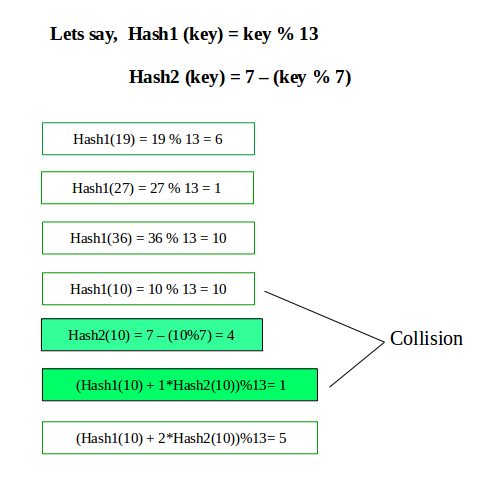# Double Hashing

Double hashing is a collision resolving technique in Open Addressed Hash tables. Double hashing uses the idea of applying a second hash function to key when a collision occurs.

Double hashing can be done using :
(hash1(key) + i * hash2(key)) % TABLE_SIZE
Here hash1() and hash2() are hash functions and TABLE_SIZE
is size of hash table.
(We repeat by increasing i when collision occurs)

First hash function is typically hash1(key) = key % TABLE_SIZE

A popular second hash function is : hash2(key) = PRIME – (key % PRIME) where PRIME is a prime smaller than the TABLE_SIZE.

A good second Hash function is:

• It must never evaluate to zero
• Must make sure that all cells can be probed`// CPP program to implement double hashing ` `#include ` `using` `namespace` `std; ` ` `  `// Hash table size ` `#define TABLE_SIZE 13 ` ` `  `// Used in second hash function. ` `#define PRIME 7 ` ` `  `class` `DoubleHash ` `{ ` `    ``// Pointer to an array containing buckets ` `    ``int` `*hashTable; ` `    ``int` `curr_size; ` ` `  `public``: ` ` `  `    ``// function to check if hash table is full ` `    ``bool` `isFull() ` `    ``{ ` ` `  `        ``// if hash size reaches maximum size ` `        ``return` `(curr_size == TABLE_SIZE); ` `    ``} ` ` `  `    ``// function to calculate first hash ` `    ``int` `hash1(``int` `key) ` `    ``{ ` `        ``return` `(key % TABLE_SIZE); ` `    ``} ` ` `  `    ``// function to calculate second hash ` `    ``int` `hash2(``int` `key) ` `    ``{ ` `        ``return` `(PRIME - (key % PRIME)); ` `    ``} ` ` `  `    ``DoubleHash() ` `    ``{ ` `        ``hashTable = ``new` `int``[TABLE_SIZE]; ` `        ``curr_size = 0; ` `        ``for` `(``int` `i=0; i "` `                     ``<< hashTable[i] << endl; ` `            ``else` `                ``cout << i << endl; ` `        ``} ` `    ``} ` `}; ` ` `  `// Driver's code ` `int` `main() ` `{ ` `    ``int` `a[] = {19, 27, 36, 10, 64}; ` `    ``int` `n = ``sizeof``(a)/``sizeof``(a); ` ` `  `    ``// inserting keys into hash table ` `    ``DoubleHash h; ` `    ``for` `(``int` `i = 0; i < n; i++) ` `        ``h.insertHash(a[i]); ` ` `  `    ``// display the hash Table ` `    ``h.displayHash(); ` `    ``return` `0; ` `} `

Output:

```0
1 --> 27
2
3
4
5 --> 10
6 --> 19
7
8
9
10 --> 36
11
12 --> 64
```

My Personal Notes arrow_drop_upCheck out this Author's contributed articles.

If you like GeeksforGeeks and would like to contribute, you can also write an article using contribute.geeksforgeeks.org or mail your article to contribute@geeksforgeeks.org. See your article appearing on the GeeksforGeeks main page and help other Geeks.

Please Improve this article if you find anything incorrect by clicking on the "Improve Article" button below.

Article Tags :
Practice Tags :

14

Please write to us at contribute@geeksforgeeks.org to report any issue with the above content.